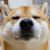# 一个有用的PHP二维数组排序函数(array_multisort)

16-12-16 00:41 字数 1830 阅读 6377 已编辑

php中的二维数组排序函数`array_multisort`刚好能解决这个问题，看下官方说明:

array_multisort — 对多个数组或多维数组进行排序
bool array_multisort ( array &\$arr [, mixed \$arg = SORT_ASC [, mixed \$arg = SORT_REGULAR [, mixed \$... ]]] )
array_multisort() 可以用来一次对多个数组进行排序，或者根据某一维或多维对多维数组进行排序。

Example1Example2Example3``````/*
* @二维数组排序 by saonian
* @param \$arr(必须) Array 要排序的数组
* @param \$field1(必须) String 数组的某一个字段
* @param \$field2(可选) String 数组的某一个字段
* @param \$sort1 Int \$field1排序规则 升序(4)or降序(3) 默认降序
* @param \$sort2 Int \$field2排序规则 升序(4)or降序(3) 默认降序
* @return Array 排序后的数组
*/
function array2_sort(\$arr,\$field1,\$field2='',\$sort1='desc',\$sort2='desc'){

if(in_array(\$sort1,array('desc','asc'))){
\$arr_sort1 = \$sort1 == 'desc' ? SORT_DESC : SORT_ASC;
}
if(in_array(\$sort2,array('desc','asc'))){
\$arr_sort2 = \$sort2 == 'desc' ? SORT_DESC : SORT_ASC;
}
\$list = array();
if(!is_array(\$arr) || \$field1 == ''){
return \$list;
}
\$arr2 = array();
foreach(\$arr as \$k => \$v){
if(!is_array(\$v) || !isset(\$v[\$field1])){//不是二维数组,或者没找到对应的元素
break;
}else{
\$arr2[] = \$v[\$field1];
}
}
if(!empty(\$field2)){
\$arr3 = array();
foreach(\$arr as \$k => \$v){
if(!is_array(\$v) || !isset(\$v[\$field2])){//不是二维数组,或者没找到对应的元素
break;
}else{
\$arr3[] = \$v[\$field2];
}
}
}
if(!empty(\$arr3)){
array_multisort(\$arr2, \$arr_sort1, \$arr3, \$arr_sort2, \$arr);
}else{
array_multisort(\$arr2, \$arr_sort1, \$arr);
}

return \$arr;
}``````

``````//comment_res:从mysql中按时间查询出的数组，并且计算好了楼层号
//up:点赞数量[第一个排序参数]
//desc 按up降序[从大到小]1人点赞>
11 条评论315

21

144

22